US: Tel: 1-206-279-3300
EU: Tel: +30 6986 007 252
• FREE VERSION OF RCsolver - Concrete Design with EC2, EC8, and ACI 318

Get full capabiltiies with our free version of RC-Solver once you signup for our newsletter. With the free version you will have to wait 30 seconds for advertisements.

# CONCRETE FRAME EXAMPLE

A concrete frame will be analized with RCsolver software program according to Eurocode 8 specifications. The frame in this example will consist of two rectangular columns and the connecting T-Section beam. RCsolver will perform capacity design of the two joints and calculate the required longitudinal and shear reinforcement for all members. The following data is available:

a. Left and right column section properties:

Rectangular section, b=0.5 m, h=0.6 m, C = 50 mm, Concrete C25/30, Steel B500C, minimum longitudinal bar diameter D14, minimum shear reinforcement bar diameter D10, number of stirrup legs = 4.

b. Left column properties:

H = 3 m, M,topz = 100 KNm, M,topy = 80 KNm, M,botz = -90 KNm, M,boty = -90 KNm, Vz = -70 KN, Vy = 80 KN, Ntop = 400 KN, Nbot = 450 KN (for axial → + for compression).

c. Right column properties:

H = 3 m, M,topz = -130 KNm, M,topy = -120 KNm, M,botz = 110 KNm, M,boty = 100 KNm, Vz = 60 KN, Vy = 70 KN, Ntop = 450 KN, Nbot = 500 KN (for axial → + for compression).

d. Beam section properties:

Beff = 1 m, h = 0.65 m, Bw = 0.25 m, tf = 0.2 m, C = 50 mm, Concrete C25/30, Steel B500C, proposed longitudinal bar diameter D18, proposed shear reinforcement diameter D10.

e. Beam properties:

L = 3 m, Mleft = -110 KNm, Mright = -120 KNm, Mspan = 70 KNm, Vleft(G+0.3Q) = -90 KN, Vright(G+0.3Q) = 90 KN, Vleft(1.35G+1.5Q) = 100 KN, Vright(1.35G+1.5Q) = -100 KN.

f. Eurocode 8 properties:

Behavior factor q = 3.9, Fundamental periods of vibration in the horizontal and in the perpendicular direction of interest: 1 sec.

Objective: Design all concrete frame members, calculating the required longitudinal and shear reinforcement.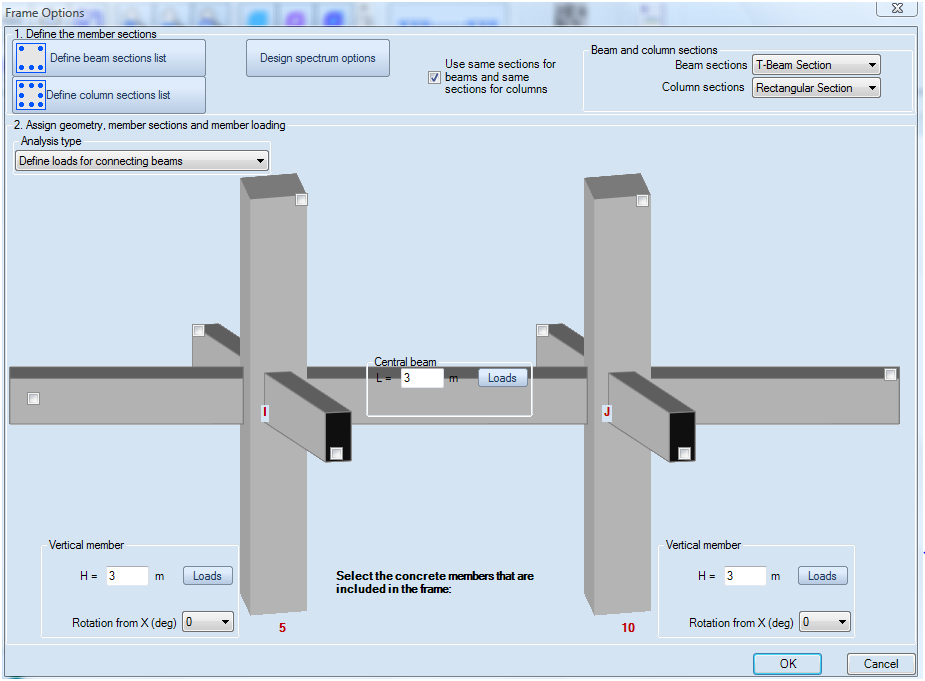Figure 1: RCsolver form for the definition of the concrete frame members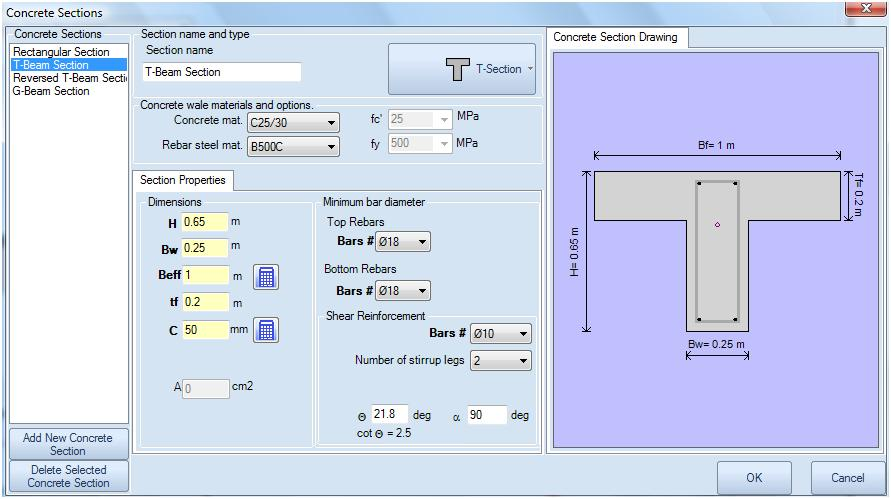Figure 2: Beam section properties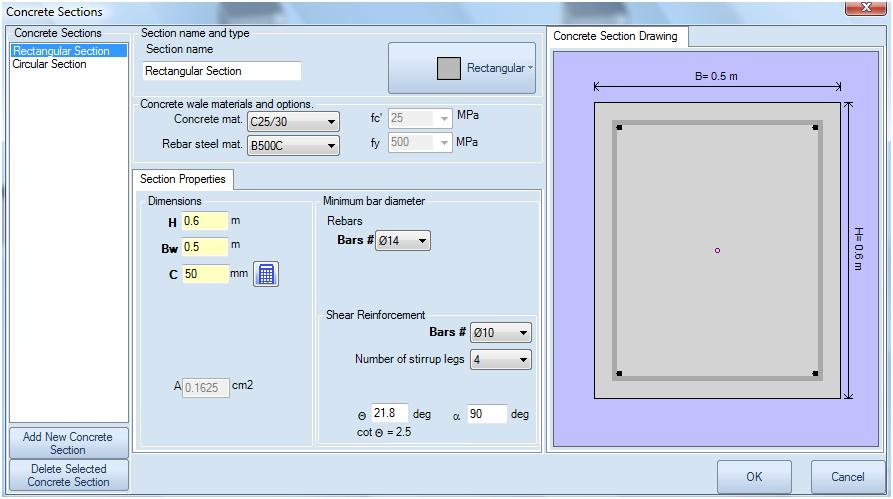Figure 3: Column section properties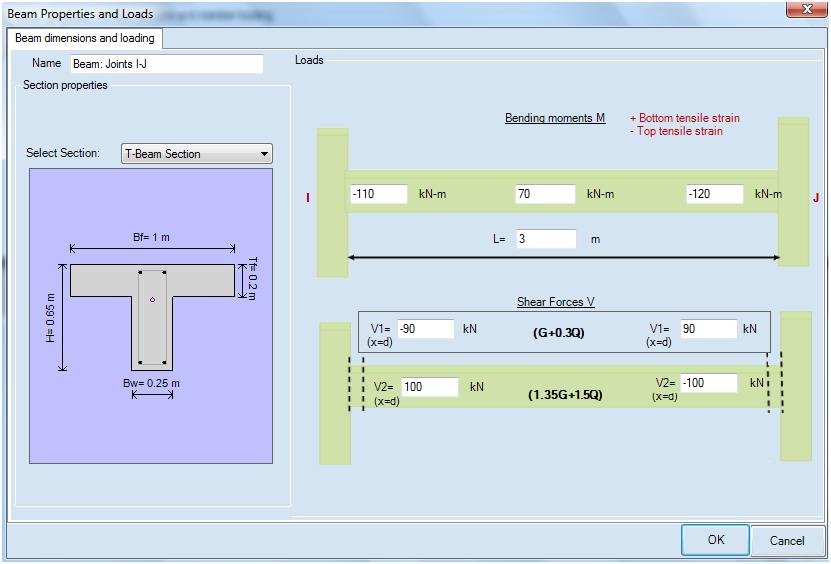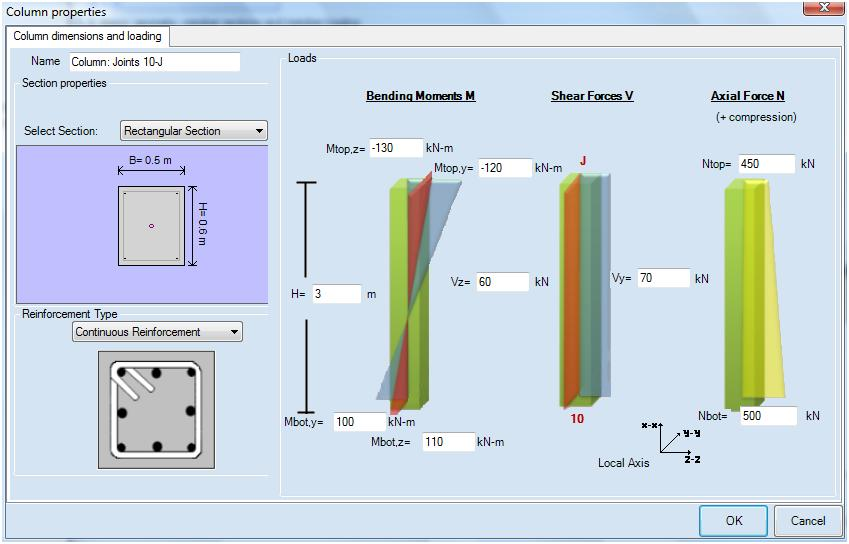Figure 7: 3D model in RCsolverFigure 8: Beam results in RCsolver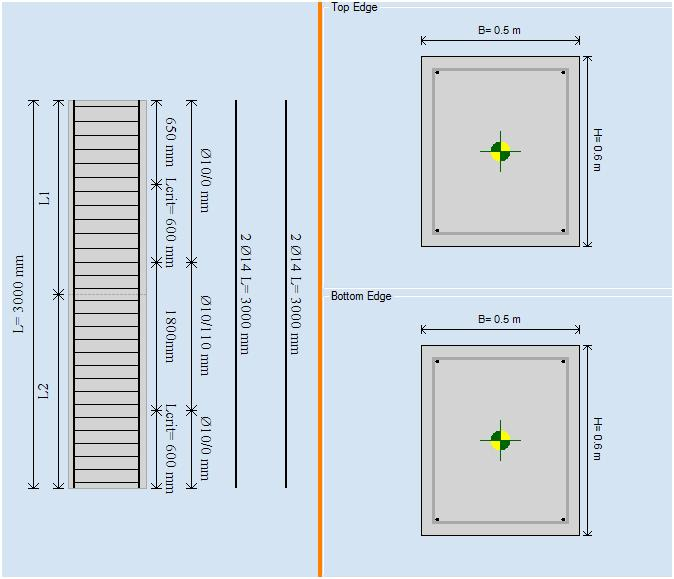Figure 9: Left column results in RCsolverFigure 10: Left column results in RCsolver

Calculations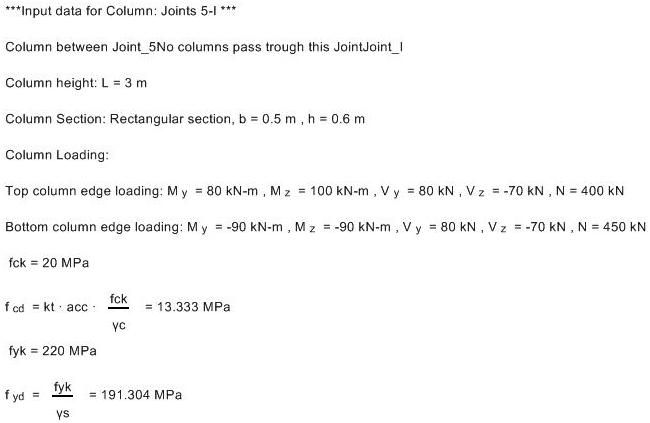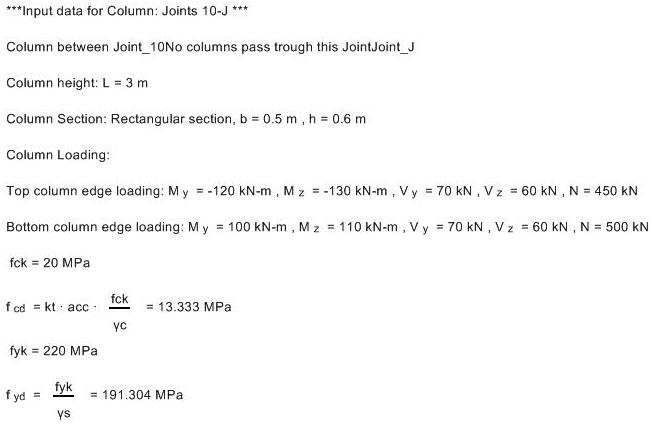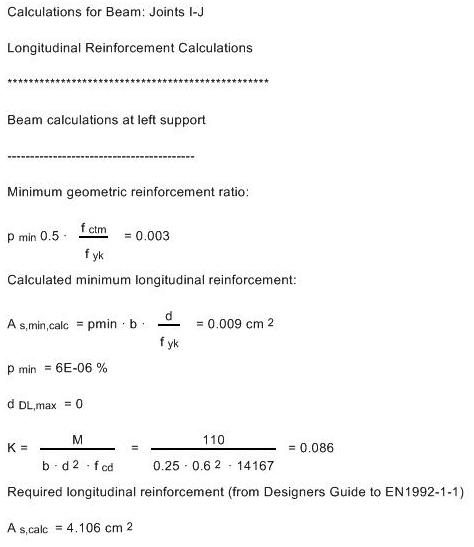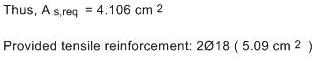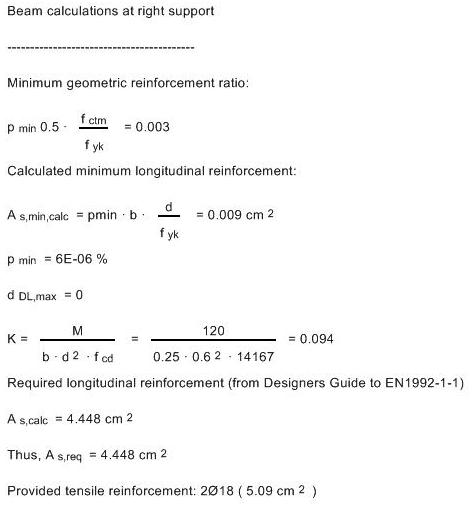* * * * * * * * * * * * * * * * * * * * * * * * * * * * * * * * * * * * * * * * * * * * * * * * * * * * * * * *

RCsolver contains all calculation procedure according to Eurocodes 2 and 8, feel free to download RCsolver and view all the equations!

# E-mail List Signup

Signup to our Email List for the latest information about our products, support and more.